## Uma Mohan (urm2), Sarvesh Sukhatme (sas624), Priyanka Venkatesh (pv228)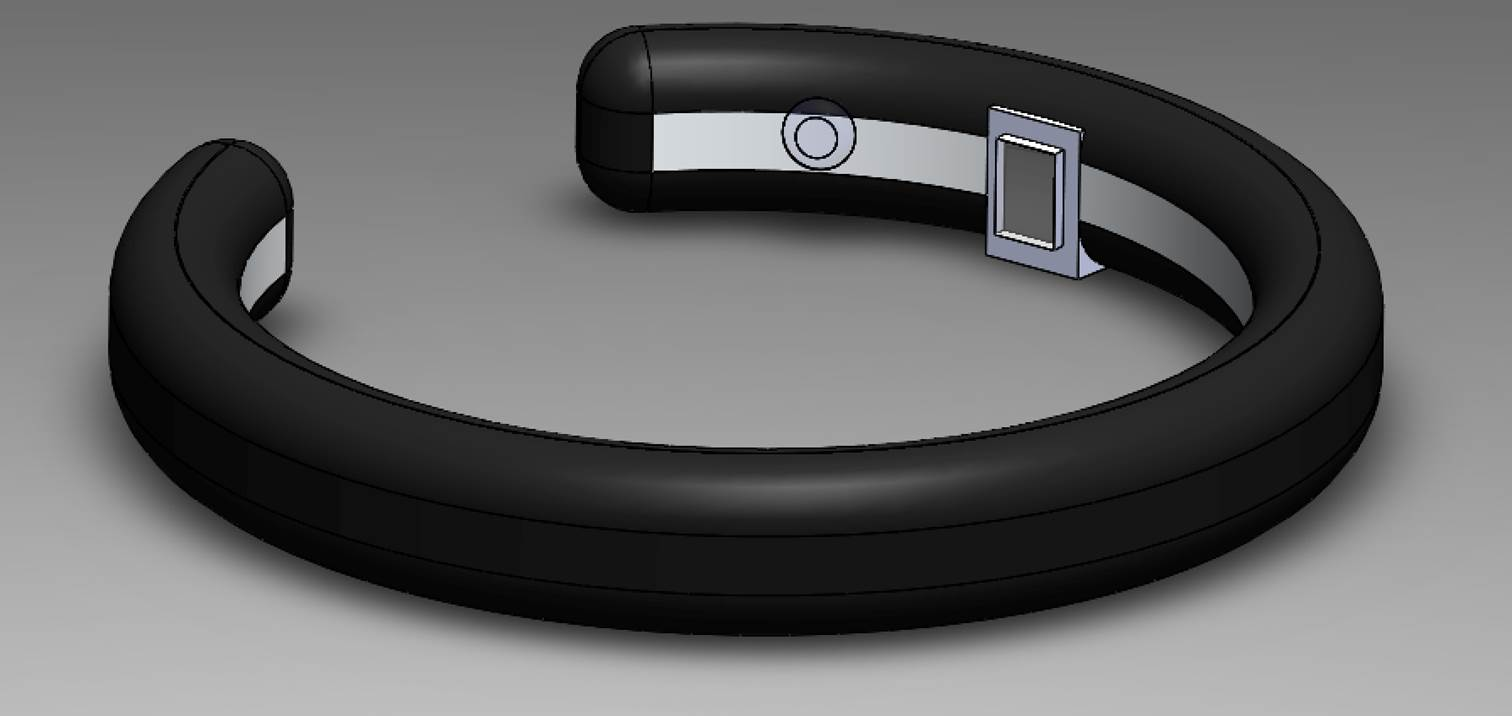Figure 1: Proposed design of wearable device detection of hydration and body fat

# Introduction and Motivation

Wearable devices have become a popular and convenient way of measuring biological metrics for fitness and healthcare. While heart rate and motion detection have been well characterized, the measurement of other parameters such as hydration and body fat have not yet been made commercially available in the form of  wearable device. The current standard for calculating total body water body fat rely on a measurement of bioelectric impedance. While this method is effective and reliable, the need to pass current through the body at two separate locations makes it difficult to make the system wearable. Thus, it is commercially available as a scale. An optical system can be used to calculate the same parameters, and by comparing optical measurements to the bioelectrical impedance measurements, this provides the redundancy needed for a reliable output. By taking advantage of the known relationship between optical density and body fat, fat percentage can be easily calculated. The challenge of relating optical density to both body fat as well as total body water can be accomplished by multivariable regression.

## Background

Bioelectrical Impedance

Bioelectrial impedance refers to the determination of the body's electrical impedance to the flow of a small electric current. This value correlates with total body water, which in turn can be used to calculate the body's fat free mass. By comparing this value with the body's total mass, the total body fat can be calculated.

Cellular tissue has both a capacitative and resistive compnent, and can be modelled as a capacitor and resistor in series. Current flows through tissue and fluids as the movement of ions. This movement is opposed by viscosity and other barriers, which can be modeled as resistance . The applied current will also charge cell membranes and various other interfaces which it comes into contacct with, which can be modelled as capacitance. The magnitude of the impedance from the series-equivalent model can be written as: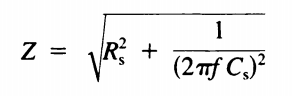Near Infrared Body Fat Measurement

Near IR interactance refers to the measurement of diffused light through a tissue from a nearby emitter source. This interactance corresponds at different wavelengths with fat and water. The peak absorption for fat is at 940 nm. The peak absorption for water is at 970 nm. Typically, the full spectrum betweek 770 and 1100 is measured, which is prohibitively large and expensive to put inside a wearable piece of technology.This device uses only one led, which emits at 940 nm. Individuals with low body fat have more muscle, which makes it difficult for light to penetrate. Similarly those with higher body fat will have less optically dense tissue, which will result in a higher measured optical density.

# Goals

Demonstrate a robust and reliable method of measuring total body fat and total body hydration using optical methods.

•Disperse near infrared wavelength  (940nm) into body.
•Measure interactance using photosensor (OPT101).
•Create regression equations using optical current measured from interactance as well as patient parameters
•Take measurements from a population of subjects  using commercially available scale utilizing bioimpedance methods.
•Calculate body fat and total body water using regression equations
•Confirm reliability of results with bioelectrical impedance measurements.
•Create GUI to display total body water and body fat percentage
•Allow GUI to take in patient metrics of age, height, weight, and gender

Future goals

•Transmit data wirelessly to app on phone.
•Keep track of body fat and total body water
•Create alert for user when either value is in unhealthy range

# Bioelectrical Impedance

The impedance is measured by passing a finite known current through the body via specific sites which depends on the parameter to be measured. The output voltage thus obtained is analyzed to get the impedance. The magnitude and phase of the signal depends on the impedance.
For our project we use BIA to obtain the body fat and skin hydration. Fat is known to be a bad conductor i.e having high resistance. Skin hydration depends on the water content of the body. Since water is a dielectric, the capacitance of the skin depends on the level of skin hydration. By measuring the impedance of the body these two parameters can be obtained
We used a LM358 Opamp to make a voltage dependent constant current source. The opamp is connected as shown in Figure 2 . Setting the resistor connected to pin2 as 200 ohms sets the current as the (voltage magnitude/200) A.  The voltage source used was a square wave with magnitude 0.2V and frequency 2Hz. The magnitude was choosen so that the resulting current is 10µA which is safe to pass through the body. A typical RC circuit has a well defined exponential decay to a voltage source. The decay depends on the characteristic time constant of the RC circuit. If the frequency of the source is comparable to the time constant of the system then the voltage saturates to the maximum value before completion of one time period. The time interval in which the output voltage reaches 0.632 of its maximum value is the time constant of the RC circuit. The resistance is simply determined by Ohm’s law as the magnitude of output voltage as well as the input current are known.
The body fat and hydration are obtained from regression equations that factor the height, weight and age of the individual along with the impedance.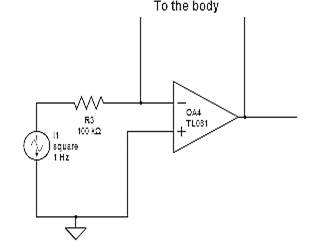Figure 2: Circuit to measure bioelectrical impedance across the user's body where one electrode is connected to the ankle and one is connected to the wrist.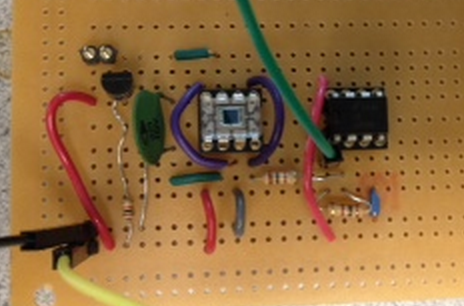Figure 3: Schamatic of constructed bioelectrical impedance circuit

# Near Infrared Measurement

Near-infrared interactance has been used as a measurement technique for estimating body fat. The interactance can be defined as the ratio of the signal obtained from a tissue to that from a reference. If E denotes the intensity then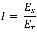Where the subscripts s & r denote the signal and reference respectively
Fat has been shown to have a maximum response to interactance at 940nm, so we used a 940nm infrared LED as the reference light source. Rather than interactance a better measure of the fat content is logarithm of the reciprocal of the interactance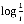OPT101 was used as a photosensor to measure the signal from the measurement site. The arrangement is such that the LED and the OPT are placed contiguously so that maximum light passes through the measurement site and has high probability of coming out near the photosensor.
The 940nm LED is driven with a constant current source made using a npn BJT. The BJT is connected as shown in Figure 4 and biased at 3.3 V to give a current of 10mA.This can be verified by measuring the voltage at the emitter of the BJT.
As the current-intensity response of the and the photodiode are linear, their ratios are a good approximation for the ratios of the intensities. Hence the interactance is calculated as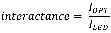The log (1/interactance) is then a variable along with height, weight, age and sex to determine the body fat (in %) and total body water (in %).
The regression equations for both methods of measurement are calibrated to a known commercially available scale .
The difference between the two methods is the characteristic variable in BIA is impedance while that in the optical method is interactance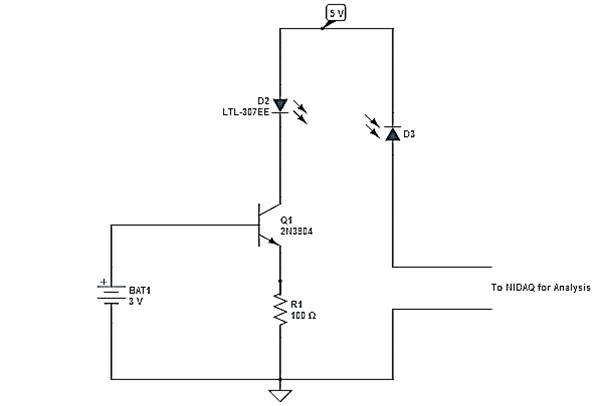Figure 4: Circuit to measure optical density utilizing 940nm IR LED and OPT101 photosensor from which optical current data is collected.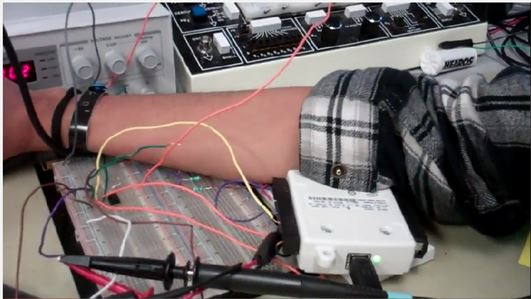Figure 5: User placing forearm such that both the LED and photosensor are directly on the same skin surface.

# Implementation

Program details:

The entire program was written by members of the group.

The National Instruments Data Acquisition device (NI-DAQ) can be used to acquire the signal output of the hardware component through a channel. This can be done by first defining the input as the NI-DAQ device.

Then the NI-DAQ must be set up as an analog input object to represent the hardware configuration.

ai = analoginput(adaptor, id);%analog input from NIDAQ

In this lab, only three channels were actually used for both the bioimpedance and optical methods; however, four signals were taken in to better understand how the input square wave signal and output bioimpedance signal compared during the process of calculating bioimpedance.

addchannel(ai, [0 1 2 3]);%four channel input

The device was configured to be used for single ended input.

set(ai, 'InputType', 'SingleEnded'); %singled ended input

In this lab, the command “getdata” was used instead of “getsample” within the main while loop to allow for 2500 samples to be taken over a second to maintain higher resolution of the signal due to more samples being able to be collected in lesser time than collecting one sample per iteration of the while loop with “get sample”.

% Obtain the available time and data as stime and asample respectively
[asample, stime] = getdata(ai, ai.SamplesPerTrigger);


Periodic Data Collection:
The device was configured with an analog sampling rate of 2500 samples per second.

% Configure the analog input rate.
set(ai, 'SampleRate', 2500); %sampling rate of 2500 sample per second 

The samples per trigger were set to 2500, which allowed a 1 second sampling period for each trigger. The data collection was set to be triggered manually with one repeat, which means that the command “getdata” must be manually used for data collection each time new data must be collected with each iteration of the while loop.

% Configure the analog input object to trigger manually.
set(ai, 'SamplesPerTrigger', 2500); % 1/2500 second
set(ai, 'TriggerRepeat', 1); %one trigger at a time
set(ai, 'TriggerType', 'manual'); %data collection occurs by manually calling getdata for each trigger 

The value of 2500 samples per trigger and a sampling rate of 2500 ensure that new data is collected periodically every second.

Graphic User Interface

1. Stop button
2. In addition, the the four graphs, the GUI includes a “Stop” button, which is used to stop the program and close the GUI. As shown here, a callback function is used to stop the program when “stopit=1”.

'callback','stopit=1;');%callback used to cause program to stop when "STOP" is pressed and changes stopit to 1 from 0

Thus, stopit is set to 0 initially, and the program is allowed to run when “stopit=0”.

while (stopit==0)
3. Total Body water and Body Fat percentages Display
4. The total body water and body fat percentages display was used to display the values of total body water and body fat percentage calculated by both bioelectrical impedance and optical methods. Both values were displayed to verify accuracy of the optical method when confirmed with bioelectrical impedance values. The display was updated with every iteration of the while loop since the variable were updated with every iteration.

5. Settable user parameters
6. The gender, age weight, and height were made to be settable by the user by using an “editable” text box. The variables sex, age, weight, and hgt, respectively, were defined as the inputted values and then used to calculate total body water and body fat. When the user inputs a value into box, the variable is updated as a string and the updated variable is used as a double (converted within the while loop) in the calculations.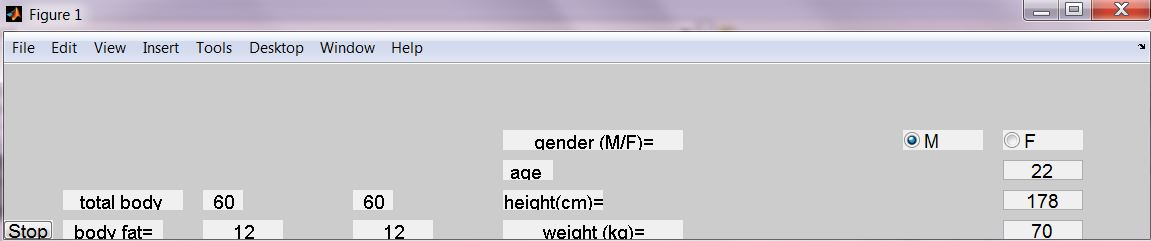Figure 6: GUI produced through MATLAB with default values.

The most challenging part of this program was developing equations that accurately calculated the body fat percentage and total body water of each subject. Equations found in literature did not produce accurate and reliable values. One possible reason is that published values did not include sufficient user parameters to accurately calculate total body water and body fat with just bioimpedance or optical current. Another reason is the change in wavelength used in this model. Furthermore, the method of calculating total body water using optical current is a novel method that was not found in literature. Thus, to overcome these challenges, multivariable regression equations were formed for both total body water and body fat using user age, gender, height, weight and the measured parameters, resistance and optical current. The function “mvregress” in MATLAB was used to correlate these parameters with known body fat and hydration values obtained through a commercially available bioelectrical impedance scale. The calculation using bioelectrical impedance fit coefficients to age, height, weight, gender, and height^2/resistance. Height was squared and divided by resistance because of the very high values of resistance calculated across the body. The calculation using optical current fit coefficients to age, height, weight, gender, and the ratio of optical currents. A coefficient was fit to the ratio of optical currents since this was used as a measure of interactance. Since gender was defined in binary in the program (male = 1, female = 0), the coefficient for gender acts has no effect on females, and it increases hydration and decreasing body fat for males by the given coefficient.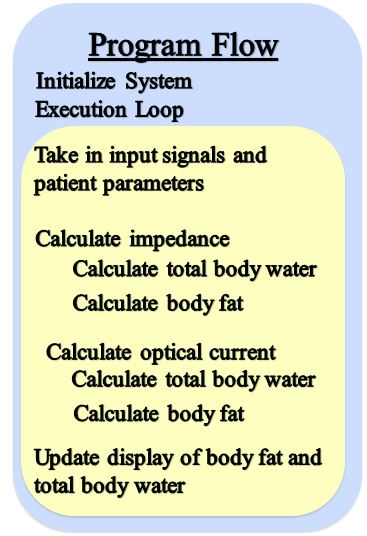Regression Equations:

Male regression equations:

Body Fat Percentage = 0.2577*age - 0.1120*height + 0.5073*weight + 3.7705*log(1/optical current) – 14.1437

Total body water percentage =  0.6986*age + 0.4140*height - 0.4919*weight + 2.6389 *log(1/optical current) + 14.6143

Female regression equation:

Body Fat Percentage = 0.2577*age - 0.1120*height + 0.5073*weight + 3.7705*log(1/optical current)

Total body water percentage=  0.6986*age + 0.4140*height - 0.4919*weight + 2.6389 *log(1/optical current)

# Results and Discussion

Regression equations were obtained by sampling 16 students; 9 male and 7 female. For each of these participants, 8 measurements were obtained including four user parameters, the measured optical current used to determine the current ratio, the resistance through the bioelectrical impedance device we built using electrodes through the wrist and ankle, and a commercial device which measures hydration and body fat through four electrodes at the feet. Each subjects gender, age, height, and weight were recorded. The commercial device took into account age, height, and mass (measured on a scale) to determine hydration and body fat. The calculations used for the commercial device were not known.

Regression equations were calculated individually for the optical body fat and hydration (see equations 1 and 2 above). The probability that the data obtained is accurate (1- p value) is 96.5% and 95.1% for body fat and hydration respectively.

Since the near infrared methods of determining body fat and hydration are significantly unique from the bioelectrical impedance methods, we were pleased to see that there were similar measurements made from the IR device compared to the commercial BI device. The sum of squares between measurements obtained from optical methods and bioelectrical impedance methods individually were 0.002 and 0.003 for hydration and body fatm respectively.

All of the participants sampled to create the regression equations and perform statistical analysis were students between the ages of 20 and 24. As a result, our regression equations may not take into account age very accurately, and our error represents accuracy within a specific population. For optical density, skin of older users appears to be thinner and less elastic due to the loss of collagen and elastin in the skin making it less difficult for light to pass through which would affect our interactance measurement. Older subjects also tend to have a slightly higher impedance measurements, possibly again due to an increase in dense, resistive body tissues.

Safety of the design was enforce by only allowing 10µA to pass through the body when taking bioelectrical impedance measurements. Additionally, all other components were electrically isolated from the user, and the intensity of the IR LED is not damaging to the skin or eyes. There was no interference between our design and other designs.

Currently, the device is simple to use with two electrodes and placing one's arm over the optical circuit; however, in future iterations of the design usability will require little consciousness of arm placement over the LED and sensor and electrode placement. This can be seen in the prototype in Figure 1 at the top of the page.

In this project we were able to successfully set up two unique, redundant methods of measuring both body fat and hydration and compare these measurements to a commercial device.With two variables each for body fat and hydration we hope to obtain a highly precise measurement of both body fat and hydration measurements. An accurate and precise hydration in particular could prove to be very valuable for athletes such as marathon runners, and patients who are suffering from hydration or otherwise need this vital to be closely monitored.

# Conclusions

The closeness of the bioelectrical impedance results and optical results was expected among subjects within the age range of 20-24, and the lack of accuracy outside of this age range was also expected. With a more substantial data set with greater diversity in parameters, the regression equations used can be improved to make the system more reliable. By using 4 patient parameters in conjunction with the measured parameters through the devices, the accuracy of calculation increased. In future studies, it would be beneficial to experiment with which frequency causes the maximum response in water to interactance. Additionally, the use of organic LEDs in future studies would advance the wearability of the device. Future goals also include making the device truly wearable by transmitting the data wirelessly to a cell phone, where the appropriate calcualtions can be made and values displayed. Ideally, this app could also keep track of a user's information and alert the user when either body fat or hydration is in an unhealthy range.

## Intellectual Property Considerations

Code and Design reuse
The previous structure of MATLAB code from previous EMG and ECG labs were used to set up data aquision and the GUI. No additional code or design was used.

Reverse-engineering
We did reverse engineer the near infrared apparatus patented in US4850365. Our system, however, uses a unique wavelength, physical configuration, and calculation method. Additionally, this apparatus was used only for acquiring a body fat percentage. Since, elements of design, utility, and methods are unique from the patented apparatus, legal implications are avoidable. According to patent law, any application filed on or after June 8, 1995, the patent term is 20 years from the filing date of the earliest U.S. application to which priority is claimed. On the other hand, for applications filed before that date, the patent term was 17 years. Therefore, this patent is now in the public domain since it was filed before 1995 in the US and its term was over 17 years.

Patent opportunities
With further data collection to refine regression equations and prototyping of the final design configuration, the physical design, calculation methods, and utility are patentable claims of the device.

Legal considerations
Our hardware does not require legal clearance, and no non-disclosure agreements were signed to get sample parts.

# Appendices

Commented Code

## Appendix B:

Schematics included in individual Bioelectrical Impedance and Near Infrared Measurement sections.

## Appendix C:

Uma Mohan - Software design
Sarvesh Sukhatme- Circuit design
Priyanka Venkatesh- Prototype design

Each of us were involved in every aspect of the project, thus making these divisions of labor descriptions of general strengths rather than strongly defined roles.

## References Used

1.Kyle, Ursula G., et al. "Bioelectrical impedance analysis—part I: review of principles and methods." Clinical Nutrition 23.5 (2004): 1226-1243.

2.Conway, Joan M., Karl H. Norris, and C. E. Bodwell. "A new approach for the estimation of body composition: infrared interactance." The American journal of clinical nutrition 40.6 (1984): 1123-1130.

3. Foster, Kenneth R., and Henry C. Lukaski. "Whole-body impedance--what does it measure?." The American journal of clinical nutrition 64.3 (1996): 388S-396S.

4. Lukaski, Henry C., et al. "Assessment of fat-free mass using bioelectrical impedance measurements of the human body." The American journal of clinical nutrition 41.4 (1985): 810-817.

5. Durnin, J. V. G. A., and J. V. G. A. Womersley. "Body fat assessed from total body density and its estimation from skinfold thickness: measurements on 481 men and women aged from 16 to 72 years." British Journal of Nutrition 32.01 (1974): 77-97.

## Acknowledgements

We would like thank our professor, Bruce Land, for his constant support and encouragement to pursue such a unique project. We would also like to thank our TA, Roland Krieger, for his guidance.